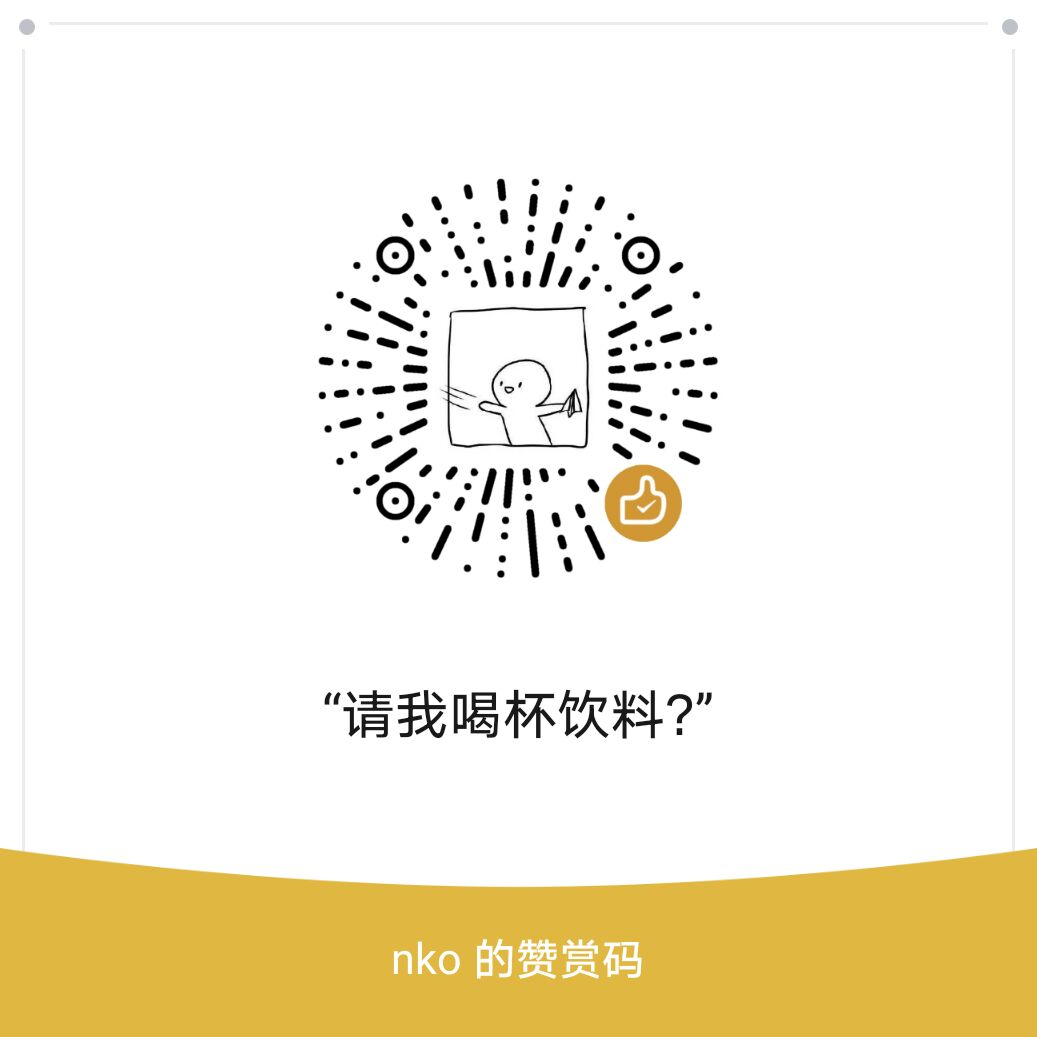# 性质

$right$集合就是代表的所有子串出现的那些位置的右端点，每一个节点所表示的那些子串的$right$集合相等

$len$表示的是当前节点的最长长度，当前节点的子串长度范围是$(len[pre[u]], len[u]]$

$right$集合的大小可以通过$topo$排序求出来，实际上用桶排实现

# 广义后缀自动机

$size$表示一个节点是多少个字符串的公共子串，这个暴力更新一下就行（注意这不是$right$集合大小）

# 应用

## bzoj 1396 & bzoj 2865

1. $i \le k \le j$
2. 子串$T$只在$S$中出现过一次

## bzoj 5084

1. 在$S$后面加入字母$C$
2. 删除$S$最后一个字母

# 习题集

• 微信扫一扫